Ch 3. Fluid Kinematics Multimedia Engineering Fluids FlowDescriptions Steady &Unsteady Streamlines,Streaklines Velocity &Acceleration IrrotationalFlow
 Chapter 1. Basics 2. Fluid Statics 3. Kinematics 4. Laws (Integral) 5. Laws (Diff.) 6. Modeling/Similitude 7. Inviscid 8. Viscous 9. External Flow 10. Open-Channel Appendix Basic Math Units Basic Equations Water/Air Tables Sections Search eBooks Dynamics Fluids Math Mechanics Statics Thermodynamics Author(s): Chean Chin Ngo Kurt Gramoll ©Kurt GramollFLUID MECHANICS - THEORY

In the previous section, velocity and acceleration of fluids was discussed. However, fluids can also rotate, which is referred to as vorticity. The concepts of both vorticity and velocity potential will be introduced. The definition of irrotational flow will then be given followed by a discussion of the potential flow.

Vorticity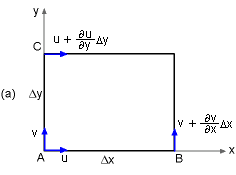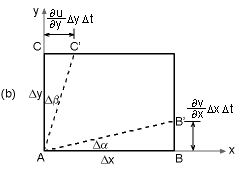Rotation of a Fluid Element
(a) At time t, and (b) At time (t + Δt)

To develop an expression that models a rotating fluid element, consider an element under rotation over a small time interval Δt as shown in the diagram on the left. Notice, points B and C can move perpendicular to to the linear flow in the x and y directions, respectively. This is cause by rotating fluid. Over a given time span, point B and C will move, as shown.

After a given time, Δt, line AB (AC will be similar) will rotate by angle Δα, giving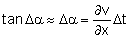where rotated angle Δα is assumed to be small. Next, the line AB will have an angular velocity, ωAB = Δα/Δt. Using Δα from above gives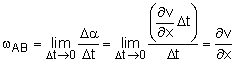Similarly, the angular velocity of the line AC is given by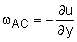The negative sign is introduced so that counter-clockwise rotation is taken to be positive.

The total angular velocity, ωz, about point A, or the z-axis, is defined as the average of the angular velocity of two mutually perpendicular lines (i.e., line AB and AC). This gives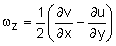Similarly, the angular velocity about the x- and y-axis are given by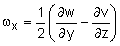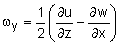GREEK "Phi" WARNING Greek Lower Case Letter Phi can be written two ways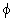or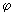(each Internet Browser does it differently). Thus,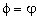Then, the rotation vector (ω) is given by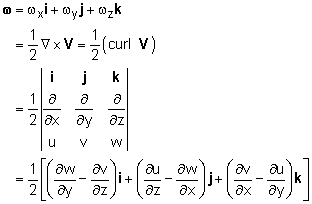where the vector operation (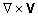) is referred to as the curl of the velocity vector V. The vorticity vector, ζ, is defined as twice the rotation vector: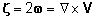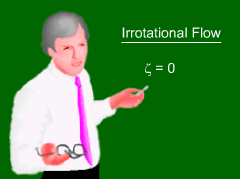Irrotational Flow

When expressed in terms of polar coordinates, the vorticity is given by: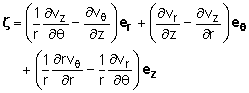A flow is said to be irrotational when there is no rotation of the fluid elements. Hence mathematically, the flow is considered irrotational when the vorticity or the curl of the velocity vanishes. That is,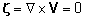Velocity Potential

 Velocity Potential Proof Expand in Cartesian coordinates,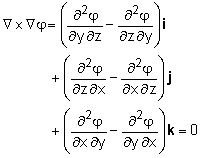The idnetity is only true for smooth function of x,y, and z.

From vector calculus, it is known that the curl of any gradient must be zero, giving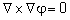This is a math identity and has nothing to do with fluids. It is just a fact. It then must be true, that a velocity potential, φ, can be introduced for irrotational flow such that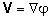While this looks like just a mathematical game, it is actually very useful. The velocity potential function, a scalar, can be used to describe the velocity field and are related to the streamlines.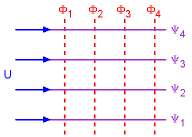Equipotential Lines and Streamlines

In Cartesian coordinates, the velocity components are related to the velocity potential as: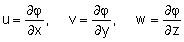In polar coordinates, they are given as: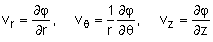Constant φ lines are referred to as the equipotential lines, and they are orthogonal to the streamlines (constant stream function ψ lines) everywhere in the flow field as shown in the diagram at the left.

Potential Flow

The concept of velocity potential is particularly useful when it is combined with the conservation of mass for incompressible fluids. For steady incompressible flow, the conservation of mass in vector form becomes the continuity equation, or simply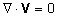If the incompressible flow is also irrotational, then the continuity equation can be written as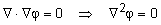The above equation is generally known as Laplace's equation, and this type of flow is referred to as potential flow. In Cartesian coordinates, the continuity equation can be expressed in terms of the velocity potential as follows: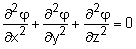Some simple plane potential flows (e.g., uniform flow, source and sink, vortex and doublet) will be introduced in the Superposition of Flows section.

Practice Homework and Test problems now available in the 'Eng Fluids' mobile app
Includes over 250 problems with complete detailed solutions.
Available now at the Google Play Store and Apple App Store.